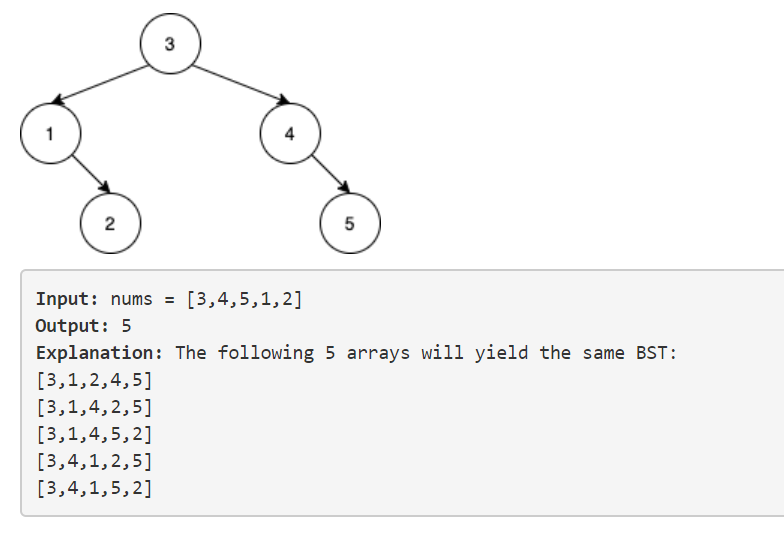## 1567. Maximum Length of Subarray With Positive Product

• 如果一个数是0，那么它不可能被包含在这个子串中，否则乘积会变成0。
• 如果一个数是正数，那么在它之前的乘积为正数的子串乘上它依然为正数，乘积为负数的子串乘上它依然为负数。
• 如果一个数是负数，那么在它之前的乘积为正数的子串乘上它会变成负数，乘积为负数的子串乘上它会变为正数。

• 使用dp[i]来表示到当前位置i最长的负乘积子串长度，使用dp[i]表示到当前位置i最长的正乘积子串长度，如果没有此种子串则长度为-1。
• 分类讨论：
1. 如果nums[i]==0，那么dp[i]=dp[i]=-1
2. 如果nums[i]>0，那么如果前面的子串长度不为-1（即存在）则可以长度+1，如果是-1（不存在）则将dp[i]重置为1；
3. 如果nums[i]<0，那么如果前面的子串长度不为-1（即存在）则可以长度+1，如果是-1（不存在）则将dp[i]重置为1；
• 需要注意的是，nums[i]<0时由于乘上负数需要变换符号，转移方程和nums[i]>0相反。

## 1568. Minimum Number of Days to Disconnect Island

• 将点$v$拆分为$v_1, v_2$，连接$w(v_1, v_2)=1$。
• 边$(u,v)$变为$(u_2,v_1)$与$(v_2,u_1)$，并$w(u_2,v_1)=w(v_2,u_1)=\infty$。
• 源点$s$，$w(s,v_1)=\infty, \forall v$ 。
• 汇点$t$，$w(v_2,t)=\infty, \forall v$ 。
• 求最小割/最大流。

## 1569. Number of Ways to Reorder Array to Get Same BST• root不变（似乎是废话）
• 左子树的元素的相对顺序不变
• 右子树的元素的相对顺序不变

• 除以左右子树的排列数后要乘上左右子树的递归解。
• 同余类下的除法需要计算逆元。
• 阶乘可以预处理。
• 开long long避免乘法溢出int32。
• 最后结果记得-1，因为询问的是还有多少种可能的方案。